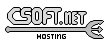Agar 1.7 Manual

M_Triangle(3)

# SYNOPSIS

```#include <agar/core.h>
#include <agar/gui.h>
#include <agar/math/m.h>
```

# DESCRIPTION

The M_Triangle2 structure describes a triangle in R2 in terms of three lines:
```typedef struct m_triangle2 {
M_Line2 a, b, c;
} M_Triangle2;
```

Similarly, M_Triangle3 describes a triangle in R3:
```typedef struct m_triangle3 {
M_Line3 a, b, c;
} M_Triangle3;
```

# INITIALIZATION

M_Triangle2 M_TriangleFromLines2 (M_Line2 L1, M_Line2 L2, M_Line2 L3)

M_Triangle3 M_TriangleFromLines3 (M_Line3 L1, M_Line3 L2, M_Line3 L3)

M_Triangle2 M_TriangleFromPts2 (M_Vector2 a, M_Vector2 b, M_Vector2 c)

M_Triangle3 M_TriangleFromPts3 (M_Vector3 a, M_Vector3 b, M_Vector3 c)

void M_TriangleWrite2 (AG_DataSource *ds, M_Triangle2 *T)

void M_TriangleWrite3 (AG_DataSource *ds, M_Triangle3 *T)

M_Triangle2 M_TRIANGLE2_INITIALIZER (M_Vector2 a, M_Vector2 b, M_Vector2 c)

M_Triangle3 M_TRIANGLE3_INITIALIZER (M_Vector3 a, M_Vector3 b, M_Vector3 c)

The functions M_TriangleFromLines2() and M_TriangleFromLines3() return an M_Triangle2 or M_Triangle3 describing a triangle in terms of three lines L1, L2 and L3. The three lines must be non-colinear and their endpoints must coincide.

M_TriangleFromPts2() and M_TriangleFromPts3() return a triangle in terms of three (non-colinear) points a, b, c.

The M_TriangleRead() and M_TriangleWrite() functions read or write a triangle structure from/to an AG_DataSource(3).

The macros M_TRIANGLE2_INITIALIZER() and M_TRIANGLE3_INITIALIZER() expand to static initializers for M_Triangle2 and M_Triangle3, respectively.

# COMPUTATIONS

int M_PointInTriangle2 (M_Triangle2 T, M_Vector2 p)

The M_PointInTriangle2() routine tests (using barycentric coordinates) whether point p lies within the triangle T and returns 1 if it does, otherwise 0.www.libAgar.org is © 2022 Julien Nadeau Carriere <vedge@csoft.net>.